## Example Questions

### Example Question #4 : How To Graph An Ordered Pair

Which of the following coordinate pairs is farthest from the origin?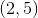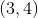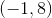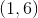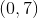Explanation:

Using the distance formula, calculate the distance from each of these points to the origin, (0, 0). While each answer choice has coordinates that add up to seven, (-1, 8) is the coordinate pair that produces the largest distance, namely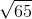, or approximately 8.06.

### Example Question #661 : Geometry

On the coordinate plane, the point with coordinates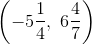is located in __________.

None of these

On the coordinate plane, a point with a negative-coordinate and a positive-coordinate lies in the upper left quadrant—Quadrant II.